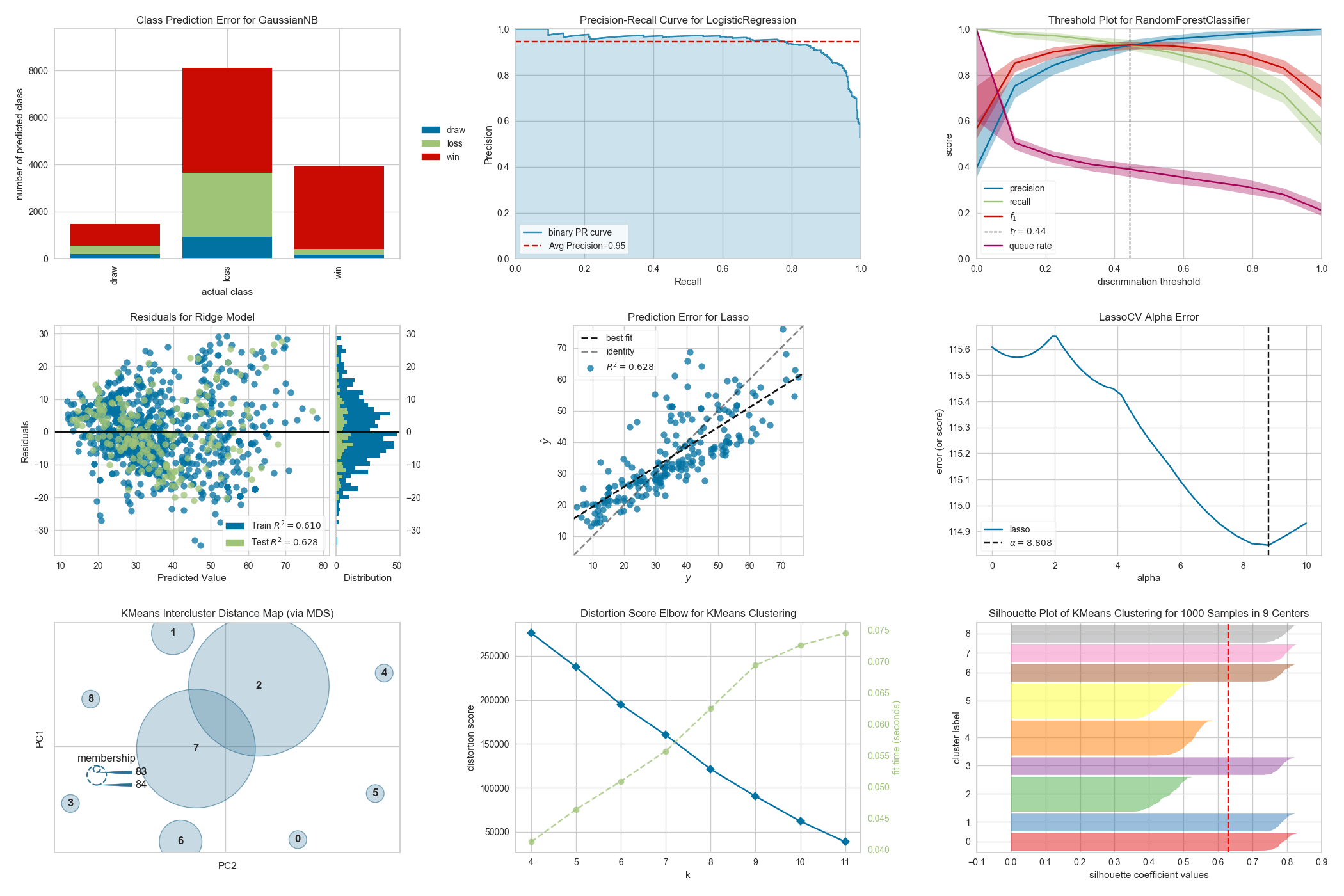# Yellowbrick; Machine Learning Visualizationhttps://github.com/DistrictDataLabs/yellowbrick/tree/develop/examples

Visualization is essential to make our analysis or modeling process understandable. We need visualization to see results or workflow, especially in machine learning algorithms.

Yellowbrick was created for this. Yellowbrick is a visualization library that can work with Scikit Learn machine learning algorithms. It is not a part of scikit-learn-contrib projects, but it uses Scikit-Learn API to make classification, clustering, hyperparameter selection, model selection, etc. It helps the user in many areas. Yellowbrick generates visualizations by wrapping Matplotlib, the most prominent Python scientific visualization library.

When we want to add a good visual to our workbook, or when we can’t decide on selection parameters or interpret scores, we can rely on Yellowbrick. Yellowbrick not only provides good visualization but also provides advice on parameters.

# Using Yellowbrick

The Yellowbrick API is specially designed to play nicely with scikit-learn. The workflow is very similar to using a scikit-learn transformer and the visualizers are intended to be integrated with scikit-learn utilities. In Yellowbrick, the primary interface is a visualizer. Visualizers are scikit-learn estimator objects.

To use the visualizers, simply use the same workflow as with a scikit-learn model. Import the visualizer, instantiate it, call the visualizer’s fit() method, then to render the visualization, call the visualizer’s show() method.

In the above example, we instantiate LinearRegression from scikit-learn. Next, for the ResidualsPlot, we instantiate the visualizer with LinearRegression from Yellowbrick. Then we use fit, score, and show methods such as scikit-learn workflow. We don’t fit LinearRegression into train data. After dividing the data, we apply Yellowbrick directly.

The image can be saved as follows In addition to the `.png` extension, `.pdf` is also commonly used for high-quality publication-ready images.

# Usage areas of Yellowbrick

• Classification Visualization
• Clustering Visualization
• Feature Visualization
• Model Selection Visualization
• Regression Visualization
• Target Visualization
• Text Visualization

We can use Yellowbrick visualizers in different areas and at different stages in the machine learning pipeline. It helps the user to make a more accurate decision with good visualization. It makes the process easier and better.

For example; Defining numbers of the cluster is a difficult choice in clustering problems. If we do not have any prior knowledge, we need to find the number of clusters for the K-mean clustering algorithm. We use the elbow method, but in this method, it is our job to choose the number of clusters. But Yellowbrick can decide instead of us with good visualization.

You can see the API documentation for Yellowbrick here.

Let’s give a few examples with Yellowbrick;

Elbow Method

In the clustering problem, we need the number of clusters and we can use the elbow method. We generate a dataset with 8 random clusters and apply KElbowVisualizer of Yellowbrick. We use the calinski_harabasz score to obtain the k parameter.

We got the plot above. It gave us the parameter k correctly. If we use the classical elbow method, our plot will be like the below. 8 seems to be the breaking point.

Prediction Error Plot

A prediction error plot shows the actual targets in the data set and the predicted values generated by our model. We can see the variance of the model. This plot also gives us the score.

Alpha Selection

Regularization is designed to penalize model complexity, therefore the higher the alpha, the less complex the model, decreasing the error due to variance (overfit). Alphas that are too high on the other hand increase the error due to bias (underfit). It is important, therefore to choose an optimal alpha such that the error is minimized in both directions.

The AlphaSelection Visualizer demonstrates how different values of alpha influence model selection during the regularization of linear models. Generally speaking, alpha increases the effect of regularization, e.g. if alpha is zero there is no regularization, and the higher the alpha, the more the regularization parameter influences the final model.

Rank Features

A two-dimensional ranking of features utilizes a ranking algorithm that takes into account pairs of features at a time (e.g. joint plot analysis). The pairs of features are then ranked by score and visualized using the lower-left triangle of a feature co-occurrence matrix.

By default, the Rank2D visualizer utilizes the Pearson correlation score to detect colinear relationships.

# Conclusion

Yellowbrick is the machine learning library that makes things easy. Making the machine learning model in Yellowbrick is not very effective because we can set parameters in scikit-learn meaningfully, but it can be used as a consultant in the visualization and machine learning process.

Yellowbrick official website has all codes you can take them and apply to your project easily.

# References

Data Scientist | Machine Learning Proficiency

## More from Hasan Ersan YAĞCI

Data Scientist | Machine Learning Proficiency